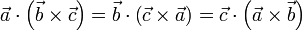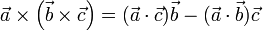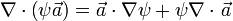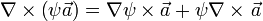# Vector identities$\vec{a} \times \vec{b} = - \vec{b} \times \vec{a}$$\vec{a}\cdot \left({\vec{b} \times \vec{c}}\right) = \vec{b}\cdot \left({\vec{c} \times \vec{a}}\right) = \vec{c}\cdot \left({\vec{a} \times \vec{b}}\right)$$\vec{a} \times \left({ \vec{b} \times \vec{c} }\right) = (\vec{a}\cdot \vec{c}) \vec{b} - (\vec{a}\cdot \vec{b}) \vec{c}$$(\vec{a} \times \vec{b})\cdot (\vec{c} \times \vec{d}) = (\vec{a}\cdot \vec{c})(\vec{b}\cdot \vec{d})-(\vec{a}\cdot \vec{d})(\vec{b}\cdot \vec{c})$$\nabla \times \nabla \psi = 0$$\nabla \cdot \left({\nabla \times \vec{a}}\right) = 0$$\nabla \times \left({\nabla \times \vec{a}}\right) = \nabla \left({\nabla \cdot \vec{a}}\right) - {\nabla }^{2}\vec{a}$$\nabla \cdot \left({\psi \vec{a}}\right) = \vec{a}\cdot \nabla \psi + \psi \nabla \cdot \left.{\vec{a}}\right.$$\nabla \times \left({\psi \vec{a}}\right) = \nabla \psi \times \vec{a} + \psi \nabla \times \left.{\vec{a}}\right.$$\nabla \left({\vec{a}\cdot \vec{b}}\right) = \left({\vec{a}\cdot \nabla }\right) \vec{b}+ \left({\vec{b}\cdot \nabla }\right) \vec{a} + \vec{a} \times \left({\nabla \times \vec{b}}\right) + \vec{b} \times \left({\nabla \times \vec{a}}\right)$$\nabla \cdot \left({\vec{a} \times \vec{b}}\right) = \vec{b}\cdot \left({\nabla \times \vec{a}}\right) - \vec{a}\cdot \left({\nabla \times \vec{b}}\right)$$\nabla \times \left({\vec{a} \times \vec{b}}\right) = \vec{a}\left({\nabla \cdot \vec{b}}\right) - \vec{b} \left({\nabla \cdot \vec{a}}\right) + \left({\vec{b}\cdot \nabla }\right)\vec{a} - \left({\vec{a}\cdot \nabla }\right)\vec{b}$